#Function Repository Resource:

# Areal

Given a simplex and point, convert back and forth between areal and Cartesian coordinates

Contributed by: Wolfram Staff
 ResourceFunction["Areal"][simplex,point] converts point between Cartesian and areal coordinates based on simplex.

## Details and Options

Areal coordinates total to one.
A simplex can be considered any of the following:
 Point [ v ] point Line [ { v 1 , v 2 } ] line segment Triangle[{v1,v2,v3}] or Polygon[{v1,v2,v3}] filled triangle Tetrahedron [ { v 1 , v 2 , v 3 , v 4 } ] filled tetrahedron Simplex [ { v 1 , v 2 , … , v n } ] an n-1 dimensional simplex

## Examples

### Basic Examples

Vertices of a 3-4-5 triangle give an example of a 2-simplex. We can convert back and forth:

 In:=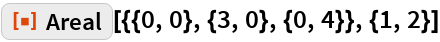Out=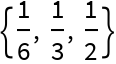In:=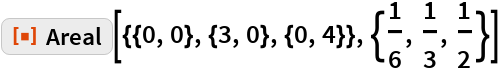Out=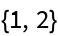Given a line segment or 1-simplex defined by two points, some other points on the line can be calculated by areal coordinates:

 In:=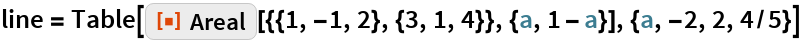Out=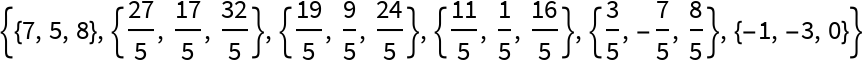Areal coordinates always have a sum of one:

 In:=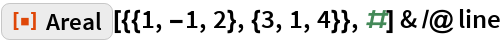Out=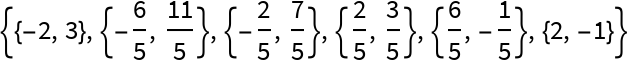### Scope

The minimal sign of an areal coordinate determines if a point is inside, outside or on the boundary of a given simplex:

 In:=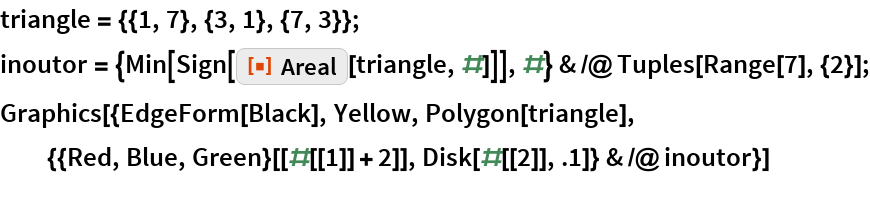Out=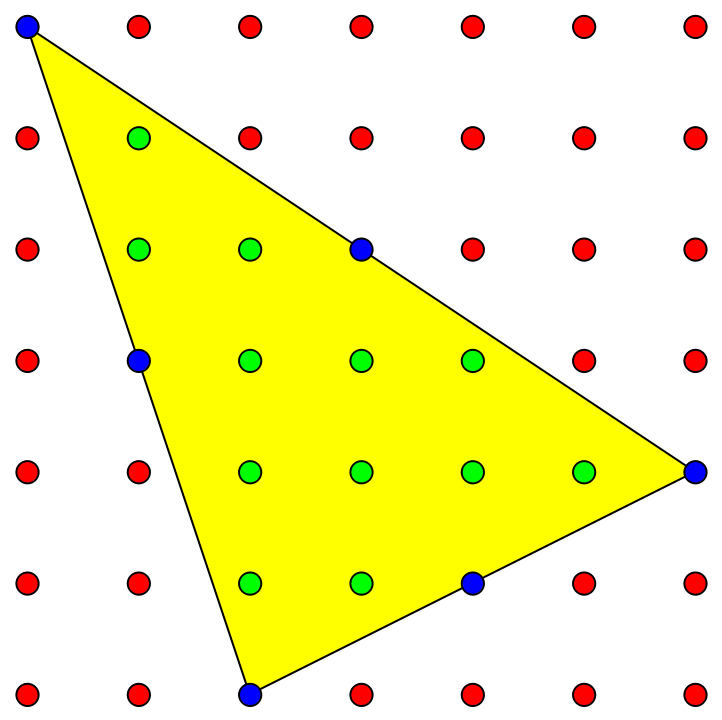By Pick’s theorem, interior+boundary/2-1=area, this triangle should have area 12+6/2-1=14:

 In:=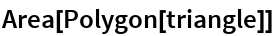Out=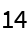Some graphics primitives that correspond to simplices are also supported:

 In:=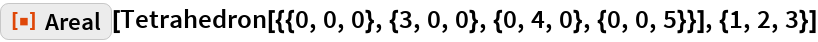Out=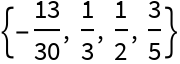The following are equivalent:

 In:=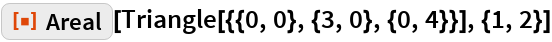Out=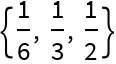In:=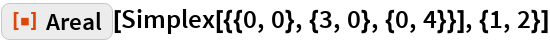Out=In:=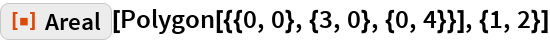Out=### Applications

The areal coordinates of the incenter are proportional to the sides of the triangle:

 In:=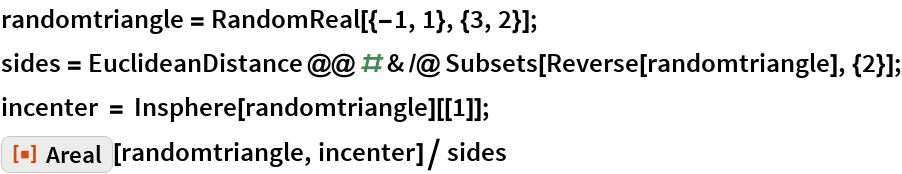Out=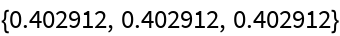The trilinear coordinate formula of a triangle center can be used with areal coordinates to generate that center; here is a calculation of the orthocenter, where the altitudes of the triangle intersect:

 In:=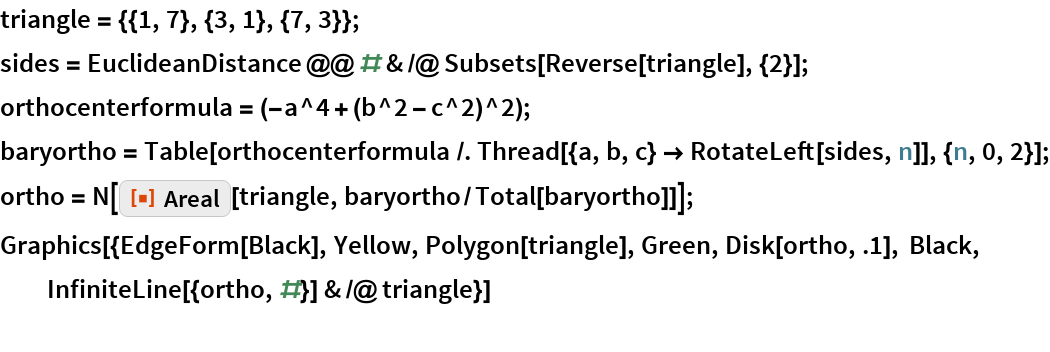Out=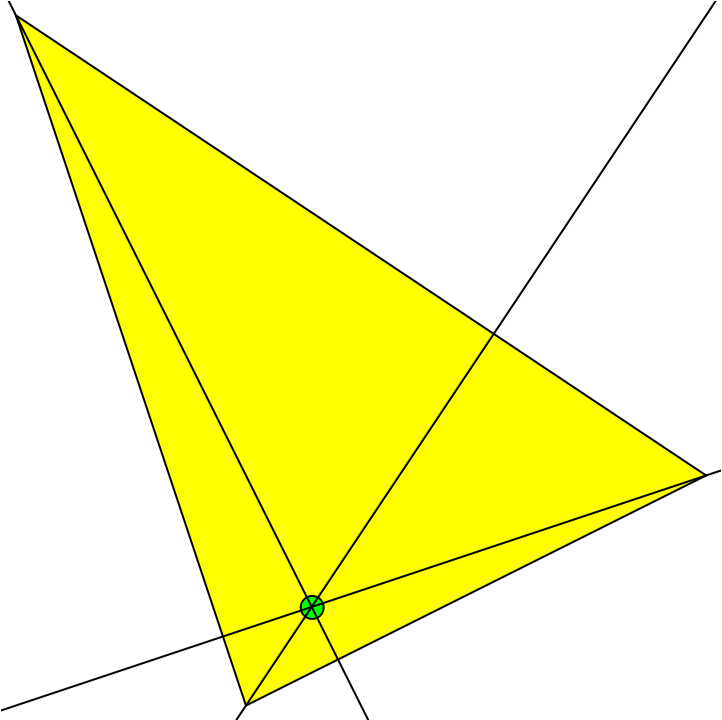### Properties and Relations

A tetrahedron is an example of a 3-simplex. We can convert back and forth:

 In:=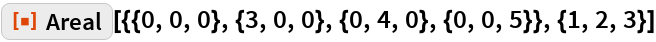Out=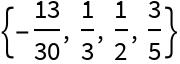In:=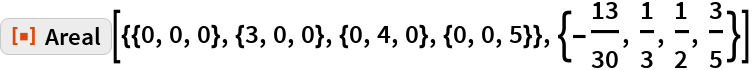Out=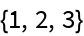The areal coordinates correspond to the area/volume when the Cartesian point is substituted for a point of the simplex and divided by the original volume; if the point is outside of the simplex, a negative value is returned:

 In:=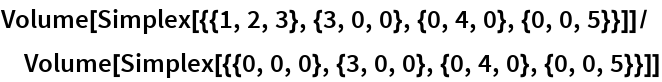Out=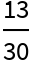### Possible Issues

The plane perpendicular to vector (1/3,1/3,1/3) has all totals equal to 1, so points on this plane are assumed to be areal coordinates and get converted to Cartesian coordinates. There exists a triangle where the areal and Cartesian coordinates are the same, but in general, there is no back function for this special case:

 In:=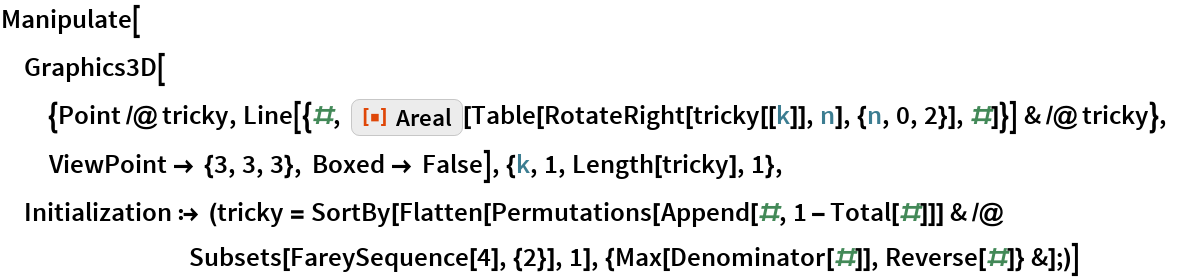Out=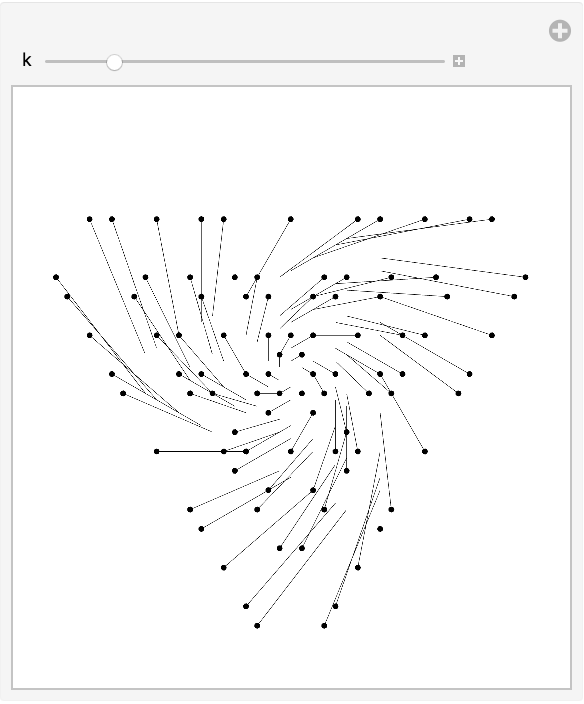### Neat Examples

Arrange 25 trees in 18 lines of five trees:

 In:=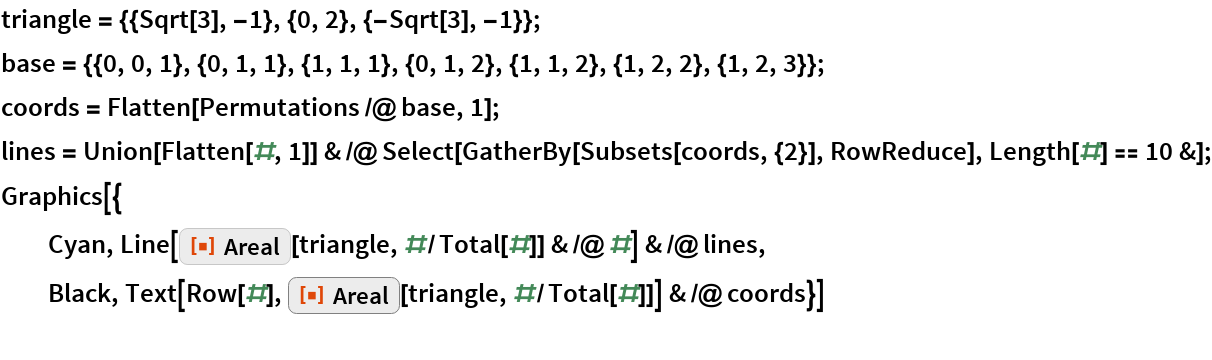Out=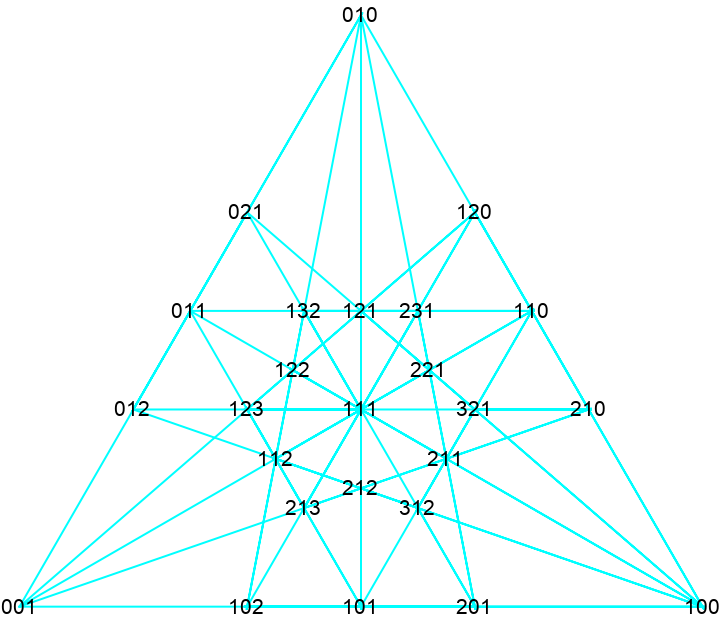Arrange 149 points in 241 lines of five:

 In:=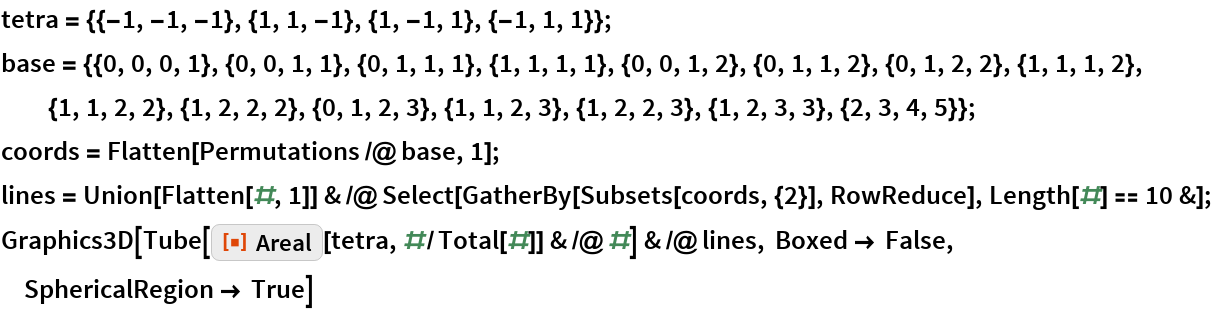Out=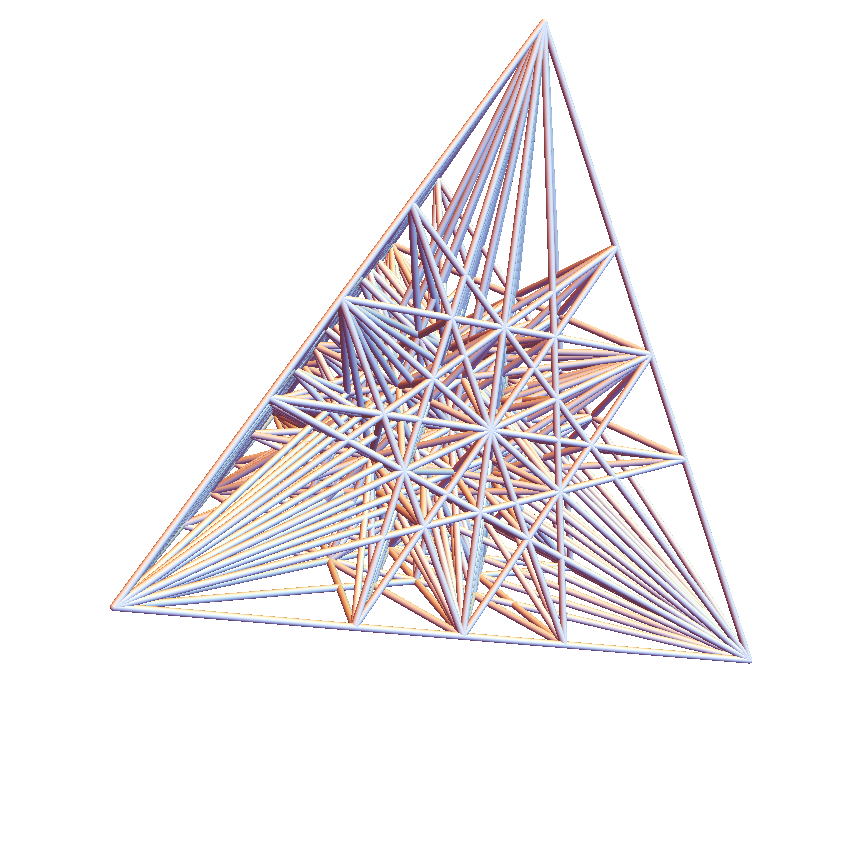## Requirements

Wolfram Language 11.3 (March 2018) or above